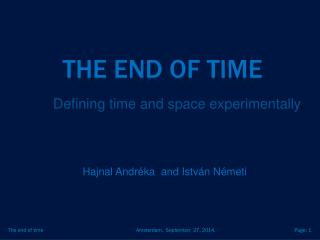DownloadDownload PresentationThe end of time

# The end of time

Télécharger la présentation## The end of time

- - - - - - - - - - - - - - - - - - - - - - - - - - - E N D - - - - - - - - - - - - - - - - - - - - - - - - - - -
##### Presentation Transcript

1. The end of time Defining time and space experimentally. Hajnal Andréka and István Németi The end of time

2. a tangible mathematical model for relationist view of Mach and Leibniz: Interpretation in the sense of math logic rich theory poor theory Theory of time Timeless theory emergence The End of Time

3. Signaling Theory SigTh Language: Send Experimenters Signals Receive James Ax’s paper “The elementary foundations of spacetime”, Foundations of Physics 8 (1978), 507-546. The End of Time

4. SigTh: Axioms all formulas valid in the intended model: Experimenters: straight lines of slope < 1 in R4 Signals: finite segments of lines of slope 1 in R4 Sends: starting point of segment lies on line Receives: end point of segment lies on line Receives(e’,s) s e’ Sends(e,s) e The End of Time

5. The End of Time

6. The End of Time

7. No clocks No meter rods The End of Time

8. The end of time

9. Special Relativity SpecRel Language: Bodies: Observers, photons Real numbers Coordinatizing World-view relation W(o,b,t,x,y,z) Mathematical world Physical world The End of Time

10. SpecRel: Four axioms Photon Axiom: the world-lines of photons are exactly the straight lines of slope 1, in each worldview Event Axiom: all observers coordinatize the same physical reality Self Axiom: the owner of a reference frame sits tight at the origin Number Axiom: the structure of our numbers forms a real closed field The End of Time

11. Thm: SpecRel can be interpreted in SigTh. Moreover, they are definitionally equivalenttheories. On the proof: Key point of interpretation is algorithm for setting up coordinate systems in terms of signaling. Comparing theories: dynamics of changing vocabularies, our paper in Johan’s Outstanding Logician Volume. The End of Time

12. What is SPACE? The End of Time

13. Motionless 1 e e e’ s’’ s e’’ s’ s’’ s’ The End of Time

14. Motionless 2 Desargue’stheorem e’ e The End of Time

15. What is TIME? The End of Time

16. Order of events Equi-duration of time-lapses Addition Multiplication. The End of Time

17. Feedback to definability theory:Defining new universes and new entities Experi- menters signals real numbers Mathematical world Physical world Defining new universes, new entities in many-sorted logic. Preprint, Andréka, H., Madarász J., Németi, I. The End of Time

18. Feedback to definability theory:Defining new universes and new entities Time? Space? experi- men- ters signals Spacetime: Minkowski geometry real numbers Mathematical world Physical world Logic and relativity (in the light of definability theory). Madarász J., PhD Dissertation, ELTE Budapest, 2002. The End of Time

19. Richard Feynman (lecture given on receiving the 1965 Nobel Prize for physics: ) Theories of the known, which are described by different physical ideas may be equivalent in all their predictions and are hence scientifically indistinguishable. However, they are not psychologically identical when trying to move from that base into the unknown. For different views suggest different kinds of modifications which might be made and hence are not equivalent in the hypotheses one generates from them in one’s attempt to understand what is not yet understood. I, therefore, think that a good theoretical physicist today might find it useful to have a wide range of physical viewpoints and mathematical expressions of the same theory (for example, of quantum electrodynamics) available to him. … there is always a range of applications and problems in this realm for which the special viewpoint gives one a special power and clarity of thought, which is valuable in itself. The End of Time

20. Other spacetimes -- General relativistic spacetime of static black hole -- Spacetime of rotating black hole -- undirected signals for EPR, faster-than-light experimenters The End of Time

21. THE END OF TIME as a primitive notion The End of Time